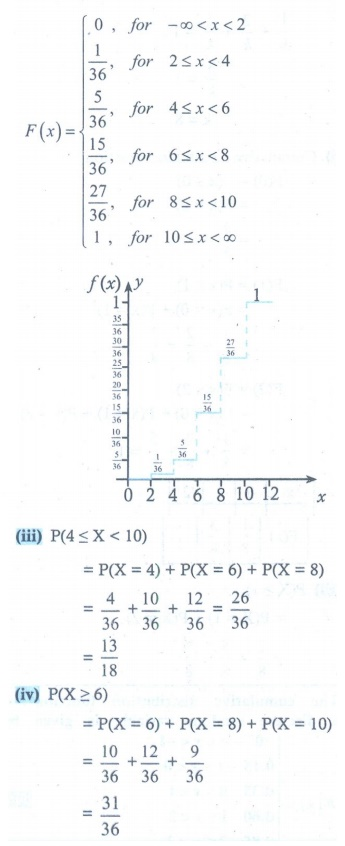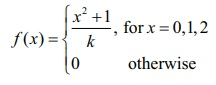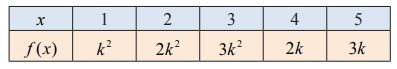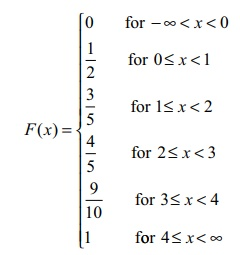Home | | Maths 12th Std | Exercise 11.2: Types of Random Variable

# Exercise 11.2: Types of Random Variable

Maths Book back answers and solution for Exercise questions - Mathematics : Probability Distributions: Types of Random Variable - Exercise Problem Questions with Answer, Solution

EXERCISE 11.2

1. Three fair coins are tossed simultaneously. Find the probability mass function for number of heads occurred.2. A six sided die is marked ‘1’ on one face, ‘3’ on two of its faces, and ‘5’ on remaining three faces. The die is thrown twice. If X denotes the total score in two throws, find

(i) the probability mass function (ii) the cumulative distribution function

(iii) P ( 4  X < 10) (iv) P ( X  6)3. Find the probability mass function and cumulative distribution function of number of girl child in families with 4 children, assuming equal probabilities for boys and girls.4. Suppose a discrete random variable can only take the values 0, 1, and 2.

The probability mass function is defined byFind (i) the value of k (ii) cumulative distribution function (iii) PX  1) .5. The cumulative distribution function of a discrete random variable is given byFind (i) the probability mass function (iiPX < 1) and (iiiPX ≥ 2) .6. A random variable x has the following probability mass function.Find (i) the value of (ii) P(2 X 5) (iii) P(3< X)7. The cumulative distribution function of a discrete random variable is given byFind (i) the probability mass function (ii) PX < 3) and (iii) PX  2) .